Prealgebra

# 9.3Use Properties of Angles, Triangles, and the Pythagorean Theorem

Prealgebra9.3 Use Properties of Angles, Triangles, and the Pythagorean Theorem

### Learning Objectives

By the end of this section, you will be able to:
• Use the properties of angles
• Use the properties of triangles
• Use the Pythagorean Theorem

### Be Prepared 9.3

Before you get started, take this readiness quiz.

1. Solve: $x+3+6=11.x+3+6=11.$
If you missed this problem, review Example 8.6.
2. Solve: $a45=43.a45=43.$
If you missed this problem, review Example 6.42.
3. Simplify: $36+64.36+64.$
If you missed this problem, review Example 5.72.

So far in this chapter, we have focused on solving word problems, which are similar to many real-world applications of algebra. In the next few sections, we will apply our problem-solving strategies to some common geometry problems.

### Use the Properties of Angles

Are you familiar with the phrase ‘do a $180’?180’?$ It means to turn so that you face the opposite direction. It comes from the fact that the measure of an angle that makes a straight line is $180180$ degrees. See Figure 9.5.

Figure 9.5

An angle is formed by two rays that share a common endpoint. Each ray is called a side of the angle and the common endpoint is called the vertex. An angle is named by its vertex. In Figure 9.6, $∠A∠A$ is the angle with vertex at point $A.A.$ The measure of $∠A∠A$ is written $m∠A.m∠A.$

Figure 9.6 $∠A∠A$ is the angle with vertex at $pointA.pointA.$

We measure angles in degrees, and use the symbol $°°$ to represent degrees. We use the abbreviation $mm$ to for the measure of an angle. So if $∠A∠A$ is $27°,27°,$ we would write $m∠A=27.m∠A=27.$

If the sum of the measures of two angles is $180°,180°,$ then they are called supplementary angles. In Figure 9.7, each pair of angles is supplementary because their measures add to $180°.180°.$ Each angle is the supplement of the other.

Figure 9.7 The sum of the measures of supplementary angles is $180°.180°.$

If the sum of the measures of two angles is $90°,90°,$ then the angles are complementary angles. In Figure 9.8, each pair of angles is complementary, because their measures add to $90°.90°.$ Each angle is the complement of the other.

Figure 9.8 The sum of the measures of complementary angles is $90°.90°.$

### Supplementary and Complementary Angles

If the sum of the measures of two angles is $180°,180°,$ then the angles are supplementary.

If $∠A∠A$ and $∠B∠B$ are supplementary, then $m∠A+m∠B=180°.m∠A+m∠B=180°.$

If the sum of the measures of two angles is $90°,90°,$ then the angles are complementary.

If $∠A∠A$ and $∠B∠B$ are complementary, then $m∠A+m∠B=90°.m∠A+m∠B=90°.$

In this section and the next, you will be introduced to some common geometry formulas. We will adapt our Problem Solving Strategy for Geometry Applications. The geometry formula will name the variables and give us the equation to solve.

In addition, since these applications will all involve geometric shapes, it will be helpful to draw a figure and then label it with the information from the problem. We will include this step in the Problem Solving Strategy for Geometry Applications.

### How To

#### Use a Problem Solving Strategy for Geometry Applications.

1. Step 1. Read the problem and make sure you understand all the words and ideas. Draw a figure and label it with the given information.
2. Step 2. Identify what you are looking for.
3. Step 3. Name what you are looking for and choose a variable to represent it.
4. Step 4. Translate into an equation by writing the appropriate formula or model for the situation. Substitute in the given information.
5. Step 5. Solve the equation using good algebra techniques.
6. Step 6. Check the answer in the problem and make sure it makes sense.
7. Step 7. Answer the question with a complete sentence.

The next example will show how you can use the Problem Solving Strategy for Geometry Applications to answer questions about supplementary and complementary angles.

### Example 9.16

An angle measures $40°.40°.$ Find its supplement, and its complement.

### Try It 9.31

An angle measures $25°.25°.$ Find its: supplement complement.

### Try It 9.32

An angle measures $77°.77°.$ Find its: supplement complement.

Did you notice that the words complementary and supplementary are in alphabetical order just like $9090$ and $180180$ are in numerical order?

### Example 9.17

Two angles are supplementary. The larger angle is $30°30°$ more than the smaller angle. Find the measure of both angles.

### Try It 9.33

Two angles are supplementary. The larger angle is $100°100°$ more than the smaller angle. Find the measures of both angles.

### Try It 9.34

Two angles are complementary. The larger angle is $40°40°$ more than the smaller angle. Find the measures of both angles.

### Use the Properties of Triangles

What do you already know about triangles? Triangle have three sides and three angles. Triangles are named by their vertices. The triangle in Figure 9.9 is called $ΔABC,ΔABC,$ read ‘triangle $ABCABC$’. We label each side with a lower case letter to match the upper case letter of the opposite vertex.

Figure 9.9 $ΔABCΔABC$ has vertices $A,B,andCA,B,andC$ and sides $a,b,andc.a,b,andc.$

The three angles of a triangle are related in a special way. The sum of their measures is $180°.180°.$

$m∠A+m∠B+m∠C=180°m∠A+m∠B+m∠C=180°$

### Sum of the Measures of the Angles of a Triangle

For any $ΔABC,ΔABC,$ the sum of the measures of the angles is $180°.180°.$

$m∠A+m∠B+m∠C=180°m∠A+m∠B+m∠C=180°$

### Example 9.18

The measures of two angles of a triangle are $55°55°$ and $82°.82°.$ Find the measure of the third angle.

### Try It 9.35

The measures of two angles of a triangle are $31°31°$ and $128°.128°.$ Find the measure of the third angle.

### Try It 9.36

A triangle has angles of $49°49°$ and $75°.75°.$ Find the measure of the third angle.

#### Right Triangles

Some triangles have special names. We will look first at the right triangle. A right triangle has one $90°90°$ angle, which is often marked with the symbol shown in Figure 9.10.

Figure 9.10

If we know that a triangle is a right triangle, we know that one angle measures $90°90°$ so we only need the measure of one of the other angles in order to determine the measure of the third angle.

### Example 9.19

One angle of a right triangle measures $28°.28°.$ What is the measure of the third angle?

### Try It 9.37

One angle of a right triangle measures $56°.56°.$ What is the measure of the other angle?

### Try It 9.38

One angle of a right triangle measures $45°.45°.$ What is the measure of the other angle?

In the examples so far, we could draw a figure and label it directly after reading the problem. In the next example, we will have to define one angle in terms of another. So we will wait to draw the figure until we write expressions for all the angles we are looking for.

### Example 9.20

The measure of one angle of a right triangle is $20°20°$ more than the measure of the smallest angle. Find the measures of all three angles.

### Try It 9.39

The measure of one angle of a right triangle is $50°50°$ more than the measure of the smallest angle. Find the measures of all three angles.

### Try It 9.40

The measure of one angle of a right triangle is $30°30°$ more than the measure of the smallest angle. Find the measures of all three angles.

#### Similar Triangles

When we use a map to plan a trip, a sketch to build a bookcase, or a pattern to sew a dress, we are working with similar figures. In geometry, if two figures have exactly the same shape but different sizes, we say they are similar figures. One is a scale model of the other. The corresponding sides of the two figures have the same ratio, and all their corresponding angles are have the same measures.

The two triangles in Figure 9.11 are similar. Each side of $ΔABCΔABC$ is four times the length of the corresponding side of $ΔXYZΔXYZ$ and their corresponding angles have equal measures.

Figure 9.11 $ΔABCΔABC$ and $ΔXYZΔXYZ$ are similar triangles. Their corresponding sides have the same ratio and the corresponding angles have the same measure.

### Properties of Similar Triangles

If two triangles are similar, then their corresponding angle measures are equal and their corresponding side lengths are in the same ratio.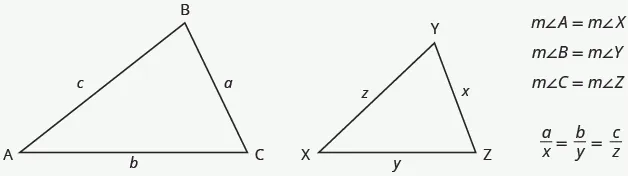The length of a side of a triangle may be referred to by its endpoints, two vertices of the triangle. For example, in $ΔABC:ΔABC:$

$the lengthacan also be writtenBCthe lengthbcan also be writtenACthe lengthccan also be writtenABthe lengthacan also be writtenBCthe lengthbcan also be writtenACthe lengthccan also be writtenAB$

We will often use this notation when we solve similar triangles because it will help us match up the corresponding side lengths.

### Example 9.21

$ΔABCΔABC$ and $ΔXYZΔXYZ$ are similar triangles. The lengths of two sides of each triangle are shown. Find the lengths of the third side of each triangle.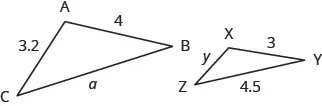### Try It 9.41

$ΔABCΔABC$ is similar to $ΔXYZ.ΔXYZ.$ Find $a.a.$### Try It 9.42

$ΔABCΔABC$ is similar to $ΔXYZ.ΔXYZ.$ Find $y.y.$### Use the Pythagorean Theorem

The Pythagorean Theorem is a special property of right triangles that has been used since ancient times. It is named after the Greek philosopher and mathematician Pythagoras who lived around $500500$ BCE.

Remember that a right triangle has a $90°90°$ angle, which we usually mark with a small square in the corner. The side of the triangle opposite the $90°90°$ angle is called the hypotenuse, and the other two sides are called the legs. See Figure 9.12.

Figure 9.12 In a right triangle, the side opposite the $90°90°$ angle is called the hypotenuse and each of the other sides is called a leg.

The Pythagorean Theorem tells how the lengths of the three sides of a right triangle relate to each other. It states that in any right triangle, the sum of the squares of the two legs equals the square of the hypotenuse.

### The Pythagorean Theorem

In any right triangle $ΔABC,ΔABC,$

$a2+b2=c2a2+b2=c2$

where $cc$ is the length of the hypotenuse $aa$ and $bb$ are the lengths of the legs.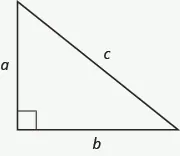To solve problems that use the Pythagorean Theorem, we will need to find square roots. In Simplify and Use Square Roots we introduced the notation $mm$ and defined it in this way:

$Ifm=n2,thenm=nforn≥0Ifm=n2,thenm=nforn≥0$

For example, we found that $2525$ is $55$ because $52=25.52=25.$

We will use this definition of square roots to solve for the length of a side in a right triangle.

### Example 9.22

Use the Pythagorean Theorem to find the length of the hypotenuse.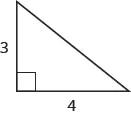### Try It 9.43

Use the Pythagorean Theorem to find the length of the hypotenuse.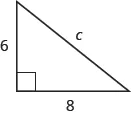### Try It 9.44

Use the Pythagorean Theorem to find the length of the hypotenuse.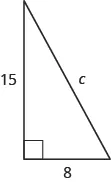### Example 9.23

Use the Pythagorean Theorem to find the length of the longer leg.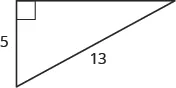### Try It 9.45

Use the Pythagorean Theorem to find the length of the leg.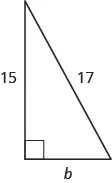### Try It 9.46

Use the Pythagorean Theorem to find the length of the leg.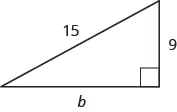### Example 9.24

Kelvin is building a gazebo and wants to brace each corner by placing a $10-inch10-inch$ wooden bracket diagonally as shown. How far below the corner should he fasten the bracket if he wants the distances from the corner to each end of the bracket to be equal? Approximate to the nearest tenth of an inch.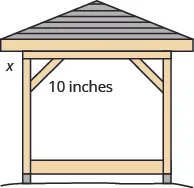### Try It 9.47

John puts the base of a $13-ft13-ft$ ladder $55$ feet from the wall of his house. How far up the wall does the ladder reach?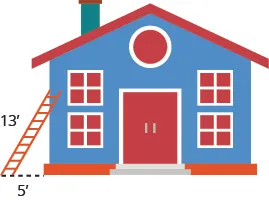### Try It 9.48

Randy wants to attach a $17-ft17-ft$ string of lights to the top of the $15-ft15-ft$ mast of his sailboat. How far from the base of the mast should he attach the end of the light string?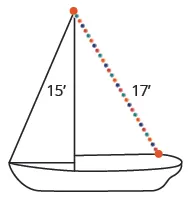### Section 9.3 Exercises

#### Practice Makes Perfect

Use the Properties of Angles

In the following exercises, find the supplement and the complement of the given angle.

81.

$53° 53°$

82.

$16° 16°$

83.

$29° 29°$

84.

$72° 72°$

In the following exercises, use the properties of angles to solve.

85.

Find the supplement of a $135°135°$ angle.

86.

Find the complement of a $38°38°$ angle.

87.

Find the complement of a $27.5°27.5°$ angle.

88.

Find the supplement of a $109.5°109.5°$ angle.

89.

Two angles are supplementary. The larger angle is $56°56°$ more than the smaller angle. Find the measures of both angles.

90.

Two angles are supplementary. The smaller angle is $36°36°$ less than the larger angle. Find the measures of both angles.

91.

Two angles are complementary. The smaller angle is $34°34°$ less than the larger angle. Find the measures of both angles.

92.

Two angles are complementary. The larger angle is $52°52°$ more than the smaller angle. Find the measures of both angles.

Use the Properties of Triangles

In the following exercises, solve using properties of triangles.

93.

The measures of two angles of a triangle are $26°26°$ and $98°.98°.$ Find the measure of the third angle.

94.

The measures of two angles of a triangle are $61°61°$ and $84°.84°.$ Find the measure of the third angle.

95.

The measures of two angles of a triangle are $105°105°$ and $31°.31°.$ Find the measure of the third angle.

96.

The measures of two angles of a triangle are $47°47°$ and $72°.72°.$ Find the measure of the third angle.

97.

One angle of a right triangle measures $33°.33°.$ What is the measure of the other angle?

98.

One angle of a right triangle measures $51°.51°.$ What is the measure of the other angle?

99.

One angle of a right triangle measures $22.5°.22.5°.$ What is the measure of the other angle?

100.

One angle of a right triangle measures $36.5°.36.5°.$ What is the measure of the other angle?

101.

The two smaller angles of a right triangle have equal measures. Find the measures of all three angles.

102.

The measure of the smallest angle of a right triangle is $20°20°$ less than the measure of the other small angle. Find the measures of all three angles.

103.

The angles in a triangle are such that the measure of one angle is twice the measure of the smallest angle, while the measure of the third angle is three times the measure of the smallest angle. Find the measures of all three angles.

104.

The angles in a triangle are such that the measure of one angle is $20°20°$ more than the measure of the smallest angle, while the measure of the third angle is three times the measure of the smallest angle. Find the measures of all three angles.

Find the Length of the Missing Side

In the following exercises, $ΔABCΔABC$ is similar to $ΔXYZ.ΔXYZ.$ Find the length of the indicated side.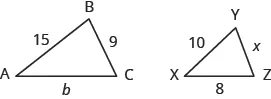105.

side $bb$

106.

side $xx$

On a map, San Francisco, Las Vegas, and Los Angeles form a triangle whose sides are shown in the figure below. The actual distance from Los Angeles to Las Vegas is $270270$ miles.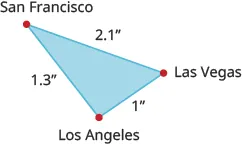107.

Find the distance from Los Angeles to San Francisco.

108.

Find the distance from San Francisco to Las Vegas.

Use the Pythagorean Theorem

In the following exercises, use the Pythagorean Theorem to find the length of the hypotenuse.

109.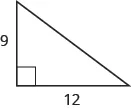110.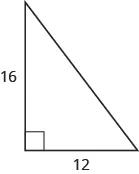111.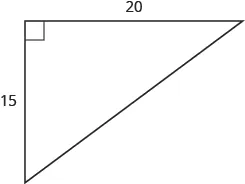112.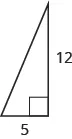Find the Length of the Missing Side

In the following exercises, use the Pythagorean Theorem to find the length of the missing side. Round to the nearest tenth, if necessary.

113.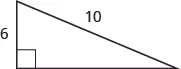114.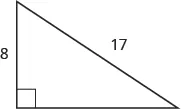115.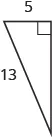116.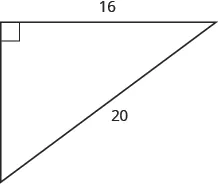117.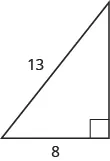118.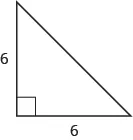119.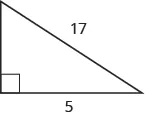120.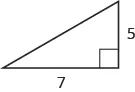In the following exercises, solve. Approximate to the nearest tenth, if necessary.

121.

A $13-foot13-foot$ string of lights will be attached to the top of a $12-foot12-foot$ pole for a holiday display. How far from the base of the pole should the end of the string of lights be anchored?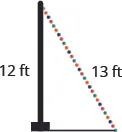122.

Pam wants to put a banner across her garage door to congratulate her son on his college graduation. The garage door is $1212$ feet high and $1616$ feet wide. How long should the banner be to fit the garage door?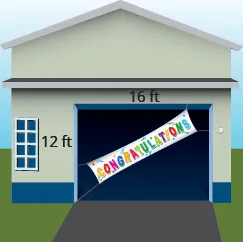123.

Chi is planning to put a path of paving stones through her flower garden. The flower garden is a square with sides of $1010$ feet. What will the length of the path be?124.

Brian borrowed a $20-foot20-foot$ extension ladder to paint his house. If he sets the base of the ladder $66$ feet from the house, how far up will the top of the ladder reach?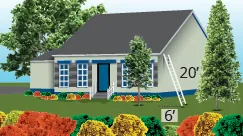#### Everyday Math

125.

Building a scale model Joe wants to build a doll house for his daughter. He wants the doll house to look just like his house. His house is $3030$ feet wide and $3535$ feet tall at the highest point of the roof. If the dollhouse will be $2.52.5$ feet wide, how tall will its highest point be?

126.

Measurement A city engineer plans to build a footbridge across a lake from point $XX$ to point $Y,Y,$ as shown in the picture below. To find the length of the footbridge, she draws a right triangle $XYZ,XYZ,$ with right angle at $X.X.$ She measures the distance from $XX$ to $Z,800Z,800$ feet, and from $YY$ to $Z,1,000Z,1,000$ feet. How long will the bridge be?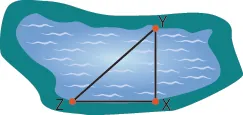#### Writing Exercises

127.

Write three of the properties of triangles from this section and then explain each in your own words.

128.

Explain how the figure below illustrates the Pythagorean Theorem for a triangle with legs of length $33$ and $4.4.$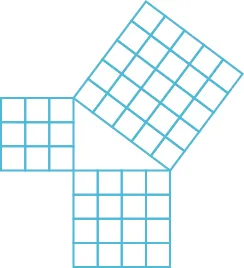#### Self Check

After completing the exercises, use this checklist to evaluate your mastery of the objectives of this section.

What does this checklist tell you about your mastery of this section? What steps will you take to improve?

Order a print copy

As an Amazon Associate we earn from qualifying purchases.